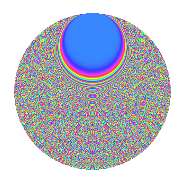# Properties

 Label 2015.2.grLevel 2015 Weight 2 Character orbit gr Rep. character $$\chi_{2015}(17,\cdot)$$ Character field $$\Q(\zeta_{60})$$ Dimension 3520 Sturm bound 448

# Related objects

## Defining parameters

 Level: $$N$$ = $$2015 = 5 \cdot 13 \cdot 31$$ Weight: $$k$$ = $$2$$ Character orbit: $$[\chi]$$ = 2015.gr (of order $$60$$ and degree $$16$$) Character conductor: $$\operatorname{cond}(\chi)$$ = $$2015$$ Character field: $$\Q(\zeta_{60})$$ Sturm bound: $$448$$

## Dimensions

The following table gives the dimensions of various subspaces of $$M_{2}(2015, [\chi])$$.

Total New Old
Modular forms 3648 3648 0
Cusp forms 3520 3520 0
Eisenstein series 128 128 0

## Trace form

 $$3520q - 18q^{2} - 10q^{3} - 48q^{6} - 24q^{7} + O(q^{10})$$ $$3520q - 18q^{2} - 10q^{3} - 48q^{6} - 24q^{7} - 12q^{10} - 48q^{11} - 18q^{12} - 14q^{13} - 30q^{15} - 420q^{16} - 4q^{17} + 42q^{18} + 90q^{20} - 96q^{21} + 40q^{22} + 2q^{23} - 16q^{25} - 48q^{26} + 20q^{27} - 30q^{28} + 120q^{30} + 30q^{32} + 18q^{33} + 12q^{35} - 1616q^{36} - 48q^{37} - 76q^{38} - 18q^{40} - 72q^{41} + 140q^{42} + 8q^{43} + 6q^{45} - 156q^{46} - 4q^{48} - 180q^{50} - 64q^{51} + 120q^{52} - 48q^{53} - 110q^{55} + 80q^{56} - 30q^{58} + 108q^{60} - 48q^{61} - 34q^{62} + 102q^{63} - 142q^{65} - 112q^{66} - 48q^{67} - 60q^{71} - 30q^{72} - 50q^{75} - 540q^{76} + 60q^{77} + 68q^{78} - 54q^{80} + 676q^{81} + 10q^{82} - 102q^{83} - 126q^{85} + 16q^{87} + 48q^{88} - 156q^{90} - 96q^{91} - 78q^{93} - 194q^{95} + 240q^{96} + 198q^{97} + O(q^{100})$$

## Decomposition of $$S_{2}^{\mathrm{new}}(2015, [\chi])$$ into irreducible Hecke orbits

The newforms in this space have not yet been added to the LMFDB.